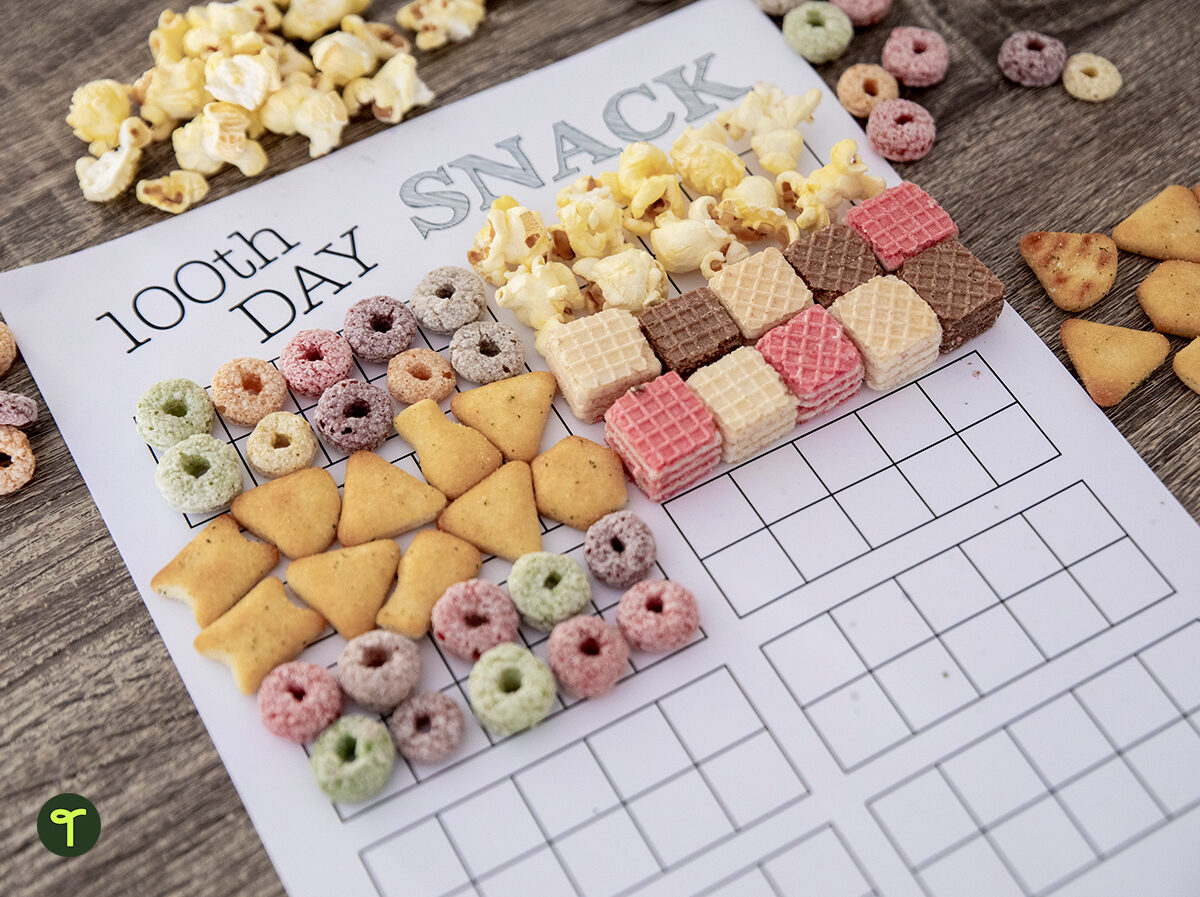Free teaching resource

100th Day Snack Template

A template for students to use to count out 100 snack items!

Why not celebrate the 100th Day of School with a snack of 100 of your favorite things?

Students will use the ten frames to count out exactly 100 pieces of a snack. Then have your students count their snacks by 2’s, 5’s, and 10’s before they eat!

Some snacks that work great for this activity are: cheerios, goldfish crackers, popcorn, and cheese puffs.Curriculum

• CCSS.MATH.CONTENT.K.CC.A.1

Count to 100 by ones and by tens.

• CCSS.MATH.CONTENT.1.NBT.A.1

Count to 120, starting at any number less than 120. In this range, read and write numerals and represent a number of objects with a written numeral.

• CCSS.MATH.CONTENT.1.NBT.B.2

Understand that the two digits of a two-digit number represent amounts of tens and ones. Understand the following as special cases:

• CCSS.MATH.CONTENT.1.NBT.B.2.A

10 can be thought of as a bundle of ten ones - called a "ten."

• CCSS.MATH.CONTENT.1.NBT.B.2.B

The numbers from 11 to 19 are composed of a ten and one, two, three, four, five, six, seven, eight, or nine ones.

• CCSS.MATH.CONTENT.1.NBT.B.2.C

The numbers 10, 20, 30, 40, 50, 60, 70, 80, 90 refer to one, two, three, four, five, six, seven, eight, or nine tens (and 0 ones).